## Numerical Integration in SQL Server, Part 2

Apr 2

Written by: Charles Flock
4/2/2014 3:23 PMIn the latest release of our math library (1.09) we introduce 5 new functions for numerical integration in SQL Server. In this second of two articles we follow up on some of the concepts behind numerical integration focusing specifically on integration over semi-infinite and infinite intervals. We will explain some of the general concepts behind numerical integration, also called quadrature, using Simpson's rule, and then look at functions for Gaussian and Double Exponential quadrature.
Recall that numerical integration or quadrature computes an approximate solution to a definite integral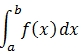to a given degree of accuracy. As before, we are only concerned with integration in one dimension. We will start off with same function that we used in Part 1.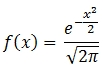We will create that function in SQL Server using the following SQL.
CREATE FUNCTION [dbo].[f]
(
@x as float
)
RETURNS float
AS
BEGIN
DECLARE @result as float

SET @result = Exp(-@x * @x / 2) / Sqrt(2 * Pi())

RETURN @result

END

GO
Let's evaluate the following semi-infinite integral: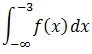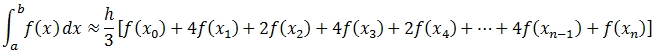But, now we have an interesting challenge in that we need evaluate the function at negative infinity. Obviously, there is no direct way to do that but it turns out that we can convert an unbounded interval into a bounded interval through the following identity.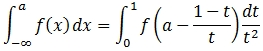Once again we can use the XLeratorDB SeriesFloat function to evaluate this transformation. The following SQL will show the values of t as well the weights (w) and the evaluation of the function f(x) using the transformed values. We will use a value of 12 for n. Remember, this illustrates the components of the calculation; the calculation itself will be in the next example.
DECLARE @a as float = -3
DECLARE @n as float = 12
DECLARE @h as float = 1e+00 / @n

SELECT
seq
,seriesvalue as t
,CASE
WHEN SEQ = 1 THEN 1
WHEN SEQ = @n+1 THEN 1
WHEN SEQ % 2 = 1 THEN 2
ELSE 4
END as w
,CASE SeriesValue
WHEN 0 THEN 0
ELSE dbo.f(@a -(1 - seriesvalue) / seriesvalue)/POWER(seriesvalue,2)
END as f
FROM
wct.SeriesFloat(0,NULL,@h,@n+1,'L')
This produces the following result.

 seq t w f 1 0.0000000000000000 1 0.00000000000000E+00 2 0.0833333333333333 4 1.57911344552012E-41 3 0.1666666666666670 2 1.81881759007328E-13 4 0.2500000000000000 4 9.72141255971726E-08 5 0.3333333333333330 2 1.33804756326087E-05 6 0.4166666666666670 4 1.43668634630708E-04 7 0.5000000000000000 2 5.35320903059542E-04 8 0.5833333333333330 4 1.18394320145775E-03 9 0.6666666666666670 2 1.96353606385296E-03 10 0.7500000000000000 4 2.74182932673974E-03 11 0.8333333333333330 2 3.43308701010937E-03 12 0.9166666666666670 4 3.99874273564903E-03 13 1.0000000000000000 1 4.43184841193801E-03

Notice that we created a CASE for t = 0 so that we avoid SQL Server generating a divide by zero error.
With a small change to our SQL, we can approximate the integral directly.
DECLARE @a as float = -3
DECLARE @n as float = 12
DECLARE @h as float = 1e+00 / @n

SELECT
@h*SUM(w*f)/3 as Integral
FROM (
SELECT
seq
,seriesvalue as t
,CASE
WHEN SEQ = 1 THEN 1
WHEN SEQ = @n+1 THEN 1
WHEN SEQ % 2 = 1 THEN 2
ELSE 4
END as w
,CASE SeriesValue
WHEN 0 THEN 0
ELSE dbo.f(@a -(1 - seriesvalue) / seriesvalue)/POWER(seriesvalue,2)
END as f
FROM
wct.SeriesFloat(0,NULL,@h,@n+1,'L')
)n
This produces the following result.
Integral
----------------------
0.00134987838244506
Let's add the exact calculation of the integral to our example and compare the results. It's beyond the scope of this article to describe the derivation of the exact integral. The beauty of numerical integration techniques is that we don't actually need to know how to come up with the limit of the anti-derivative, which is what is required in this calculation.
DECLARE @a as float = -3
DECLARE @n as float = 12
DECLARE @h as float = 1e+00 / @n

SELECT
@h*SUM(w*f)/3 as [Approximate Integral]
,0.5*(1+wct.ERF(@a/SQRT(2))) as [Exact Integral]
FROM (
SELECT
seq
,seriesvalue as t
,CASE
WHEN SEQ = 1 THEN 1
WHEN SEQ = @n+1 THEN 1
WHEN SEQ % 2 = 1 THEN 2
ELSE 4
END as w
,CASE SeriesValue
WHEN 0 THEN 0
ELSE dbo.f(@a -(1 - seriesvalue) / seriesvalue)/POWER(seriesvalue,2)
END as f
FROM
wct.SeriesFloat(0,NULL,@h,@n+1,'L')
)n
This produces the following result.
Approximate Integral   Exact Integral
---------------------- ----------------------
0.00134987838244506    0.00134989803163127
Generally we can improve upon the approximation by increasing @n. Instead of @n = 12, we set @n = 1,000 and re-run our SQL producing the following result.
Approximate Integral   Exact Integral
---------------------- ----------------------
0.00134989803163024    0.00134989803163127
With @n = 1,000 we produce a highly accurate approximation of the semi-infinite integral. However, let's see what happens when we integrate from a to infinity.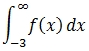Again, we can transform the unbounded interval into a bounded interval through the following identity.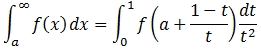Remarkably, this requires us to only change a minus sign to a plus sign in our SQL.
DECLARE @a as float = -3
DECLARE @n as float = 12
DECLARE @h as float = 1e+00 / @n

SELECT
@h*SUM(w*f)/3 as [Approximate Integral]
,1-0.5*(1+wct.ERF(@a/SQRT(2))) as [Exact Integral]
FROM (
SELECT
seq
,seriesvalue as t
,CASE
WHEN SEQ = 1 THEN 1
WHEN SEQ = @n+1 THEN 1
WHEN SEQ % 2 = 1 THEN 2
ELSE 4
END as w
,CASE SeriesValue
WHEN 0 THEN 0
ELSE dbo.f(@a +(1 - seriesvalue) / seriesvalue)/POWER(seriesvalue,2)
END as f
FROM
wct.SeriesFloat(0,NULL,@h,@n+1,'L')
)n
This produces the following result.
Approximate Integral   Exact Integral
---------------------- ----------------------
1.03670801699995       0.998650101968369
Again, we can improve upon the approximation by increasing @n. With @n = 1,000 we get the following result.
Approximate Integral   Exact Integral
---------------------- ----------------------
0.998650101968363      0.998650101968369
In our third example, we will integrate over an infinite interval.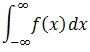As in the previous two examples we can transform this into integration over a finite interval using the following identity.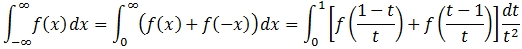DECLARE @n as float = 12
DECLARE @h as float = 1e+00 / @n

SELECT
@h*SUM(w*f)/3 as [Approximate Integral]
,1 as [Exact Integral]
FROM (
SELECT
seq
,seriesvalue as t
,CASE
WHEN SEQ = 1 THEN 1
WHEN SEQ = @n+1 THEN 1
WHEN SEQ % 2 = 1 THEN 2
ELSE 4
END as w
,CASE SeriesValue
WHEN 0 THEN 0
ELSE (dbo.f((1 - seriesvalue) / seriesvalue)+ dbo.f((seriesvalue - 1) / seriesvalue))/POWER(seriesvalue,2)
END as f
FROM
wct.SeriesFloat(0,NULL,@h,@n+1,'L')
)n
This produces the following result.
Approximate Integral   Exact Integral
---------------------- --------------
0.997726201813227      1

When we increase
@n to 1,000 we get the following result.
Approximate Integral   Exact Integral
---------------------- --------------
0.999999999999947      1
In our latest release of XLeratorDB/math and XLeratorDB/math 2008 we have included 3 new functions for numerical integration over semi-infinite and infinite intervals: QUAD, QUADDE, and QUADOSC. The techniques used in these functions are much like the techniques described above. The QUAD function uses the appropriate identities to transform that intervals and is an adaptive algorithm using Gauss 7-point and Kronrod 15-point rules.
We believe that these three quadratures are the most robust quadrature methods available for semi-infinite and infinite integrals. We have packaged them up to make the SQL as simple as possible while still providing a high degree of flexibility and performance. Let's see how they work.
If you go back to the first example, you can see that we created a user-defined function (UDF). While this was not strictly necessary, we did it to make the SQL simpler, especially with respect to integrals over infinite intervals. In QUAD, QUADDE and QUADOSC you enter the function definition as a string, giving you the option of invoking SQL Server built-in functions, user-defined functions, and SQL CLR functions, or any combination of them. Additionally if you do not provide alternate definitions for undefined regions of function evaluation (e.g. divide by zero, ln(0), gamma(-1), etc.), these functions will trap the error and set f(x) = 0 at that point.
Let's look at our first example. We would enter the following SQL using QUADDE.
SELECT
wct.QUADDE('SELECT Exp(-@x * @x / 2) / Sqrt(2 * Pi())','@x','-Inf',-3) as [Double Exponential]
,0.5*(1+wct.ERF(-3/SQRT(2))) as [Exact Integral]

This produces the following result.
Double Exponential     Exact Integral
---------------------- ----------------------
0.0013498980316301     0.00134989803163127

The
QUAD function has the same calling structure as QUADDE. The following SQL returns the results of both quadratures and compares them to the exact integral.
SELECT
wct.QUADDE('SELECT Exp(-@x * @x / 2) / Sqrt(2 * Pi())','@x','-Inf',-3) as [Double Exponential]
,wct.QUAD('SELECT Exp(-@x * @x / 2) / Sqrt(2 * Pi())','@x','-Inf',-3) as [Gaussian]
,0.5*(1+wct.ERF(-3/SQRT(2))) as [Exact Integral]

This produces the following result.
Double Exponential     Gaussian               Exact Integral
---------------------- ---------------------- ----------------------
0.0013498980316301     0.0013498980316301     0.00134989803163127

The
QUADOSC function contains an additional parameter for oscillatory functions. Since the function we are integrating is not oscillatory, we can set the oscillatory parameter to 1 and it will return the same value as QUADDE.
SELECT
wct.QUADDE('SELECT Exp(-@x * @x / 2) / Sqrt(2 * Pi())','@x','-Inf',-3) as [Double Exponential]
,wct.QUAD('SELECT Exp(-@x * @x / 2) / Sqrt(2 * Pi())','@x','-Inf',-3) as [Gaussian]
,wct.QUADOSC('SELECT Exp(-@x * @x / 2) / Sqrt(2 * Pi())','@x','-Inf',-3,1) as [Oscillatory]
,0.5*(1+wct.ERF(-3/SQRT(2))) as [Exact Integral]

This produces the following result.
Double Exponential     Gaussian               Oscillatory            Exact Integral
---------------------- ---------------------- ---------------------- -------------------
0.0013498980316301     0.0013498980316301     0.0013498980316301     0.00134989803163127

We also could have used our UDF in the numerical integration.
SELECT
,0.5*(1+wct.ERF(-3/SQRT(2))) as [Exact Integral]
This returns exactly the same result as the previous example.

Let's look at one more example.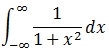SELECT
,PI() as [Exact Integral]

This produces the following result.
Double Exponential     Gaussian               Oscillatory            Exact Integral
---------------------- ---------------------- ---------------------- -----------------
3.14159265358979       3.14159265358979       3.13427314536748       3.14159265358979

Notice that
QUADDE and QUAD exactly match the exact integral while QUADOSC has only 2 digits of accuracy. This underscores the fact that there is not one all-encompassing quadrature technique that will integrate any expression. Different functions may require different approaches. We have provided 3 quadrature functions which should enable you to integrate the greatest variety of functions over semi-infinite and infinite intervals.
Download a 15-day free trial today and see how numerical integration could work for you.

Tags:
Categories:
Location: BlogsThe WestClinTech Blog

## Search Blogs

 KeywordsPhrase

## Blog Archives

Archive
 < September 2023 >
SunMonTueWedThuFriSat
272829303112
3456789
10111213141516
17181920212223
24252627282930
1234567
Monthly
Go

### SupportCopyright 2008-2023 Westclintech LLC         Privacy Policy        Terms of Service